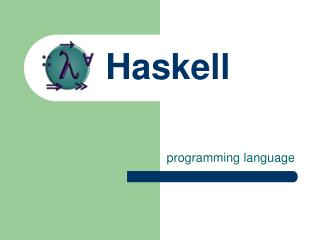Download Presentation# Haskell - PowerPoint PPT Presentation

Haskell. programming language. Haskell is…. Memory managed (allocation, collection) “Typeful” (static, strong) Types are checked at compile-time Types cannot be coerced (in general) Pure functional programming Emphasis on functions Referential transparency All variables are constant.I am the owner, or an agent authorized to act on behalf of the owner, of the copyrighted work described.
Download PresentationAn Image/Link below is provided (as is) to download presentation

Download Policy: Content on the Website is provided to you AS IS for your information and personal use and may not be sold / licensed / shared on other websites without getting consent from its author.While downloading, if for some reason you are not able to download a presentation, the publisher may have deleted the file from their server.

- - - - - - - - - - - - - - - - - - - - - - - - - - E N D - - - - - - - - - - - - - - - - - - - - - - - - - -
Presentation Transcript
1. Haskell programming language

2. Haskell is… • Memory managed (allocation, collection) • “Typeful” (static, strong) • Types are checked at compile-time • Types cannot be coerced (in general) • Pure functional programming • Emphasis on functions • Referential transparency • All variables are constant

3. Haskell - pros & cons • Concurrency • The #1 on Language Shootout for threading • All non-I/O code is concurrent by default • (mutations are handled as I/O code) • Readability • No parentheses or commas • Higher-order functions reduce lines-of-code • (no syntax as a reminder of context)

4. Main main True a Bool Eq -- modules (packages) -- value variables (functions) -- value constructors -- type variables (generics) -- type constructors -- type classes (interfaces) Haskell - namespaces

5. Derived syntax f x = expr (x *) (* y) (*) x `times` y f \$ g \$ h x (f . g . h) x do a; b; c -- lambda -- sections -- sections -- sections -- infix func -- parens -- compose -- I/O bind Haskell - syntax Core syntax f = \x -> expr \y -> x * y \x -> x * y \x y -> x * y times x y f (g (h x)) f (g (h x)) a >>= b >>= c

6. Haskell - type syntax life = 42 :: Int life :: Int life = 42

7. Haskell - types “Hello” :: String length :: [a] -> Int floor :: Float -> Int map :: (a -> b) -> [a] -> [b] 42 :: Int (+) :: Int -> Int -> Int 42 :: Num a => a (+) :: Num a => a -> a -> a

8. Int, Word, Float, Double, Char type String = [Char] data Maybe a = Nothing | Just a class Eq a where (==), (/=) :: a -> a -> Bool instance Eq Bool where True == True = True False == False = True _ == _ = False -- built-in types -- type synonyms -- data types -- type classes -- type class instances Haskell - type system

9. data Bool = False | True data Tree a = Leaf a | Branch (Tree a) (Tree a) data Rect = Rect Int Int Int Int data Rect = Rect { x, y :: Int, width :: Int, height :: Int} -- enumerations -- generics -- unlabeled record -- labeled record Haskell - datatypes

10. main = return () main = putStrLn “Hello World” main = interact id main = interact (unlines . reverse . lines) main = do args <- getArgs case args of "-n":a -> putStr (unwords a) a -> putStrLn (unwords a) -- null program -- hello world -- UNIX cat -- GNU tac -- UNIX echo Haskell - example programs

11. version control system

12. Darcs - overview • The Theory of Patches • A “branch” is a set of patches • “Spontaneous branches” • Every checkout is a branch • Every checkout is a repository

13. Darcs - interactive • Interactive “pull” • You chose what patches to download • Interactive “push” • You chose what patches to commit externally • Interactive “record” • You chose what files to commit locally

14. Parsec parser library

15. many :: Parser a -> Parser [a] many1 :: Parser a -> Parser [a] optional :: Parser a -> Parser (Maybe a) sepBy :: Parser a -> Parser s -> Parser [a] sepBy1 :: Parser a -> Parser s -> Parser [a] endBy :: Parser a -> Parser s -> Parser [a] endBy1 :: Parser a -> Parser s -> Parser [a] char :: Char -> Parser Char between :: Parser open -> Parser close -> Parser a -> Parser a -- like regex* -- like regex+ -- like regex? -- ... , ... , ... -- ... ; ... ; ... ; -- c -- < ... > Parsec - combinators

16. Parsec - example parsers number = many digit string = between (char ‘"’) (char ‘"’) (many anyChar) lisp = number <|> string <|> identifier <|> parens \$ many \$ lexeme lisp

17. xmonad X11 window manager

18. XMonad - overview • Tilling window manager (like Ratpoison) • Libraries of extensions and status bars • Customizable (config file is the app) • Full keyboard accessibility